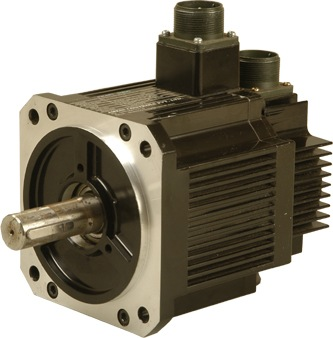May 24, 2009

# Calculating Power Required for Selecting Motor for Your ApplicationMotor selection required power rating and let us refresh our basics and see how to calculate the power required for your application.Let us say we have an application where rotating parts having moment of inertia I and need to transfer T torque at a speed of N.  And an additional design objective is it should have minimum α angular acceleration while stating our application.

Let us have a closer look at each parameters units.(Note all calculations are done in SI units)

Torque - Nm

Rotation Speed – RPM

Moment of Inertia -  N.m^2

Power - W

Now we know

Power = 2.π.N.T/60

So power needed to transmit T torque can be found from the above equation.

We also need α angular acceleration at every startups along with torque transfer.

Power required to generate α angular acceleration can be found from the following equation

P= I.α

Now Total Power required for our application is

P = I.α + 2.π.N.T/60

Now we can select a motor which can deliver power we calculated.

Note:-While calculating torque you should also consider all the friction couples in your application.